Get instant live expert help with Excel or Google Sheets“My Excelchat expert helped me in less than 20 minutes, saving me what would have been 5 hours of work!”

#### Post your problem and you’ll get expert help in seconds.

Your message must be at least 40 characters
Our professional experts are available now. Your privacy is guaranteed.

# How to Split Text and Numbers with a Formula in Excel

We can easily split text and numbers from a cell by using different combinations of functions such as FIND, MIN, LEN, LEFT, and RIGHT functions. The steps below will walk through the process of splitting text and numbers contained in a Cell.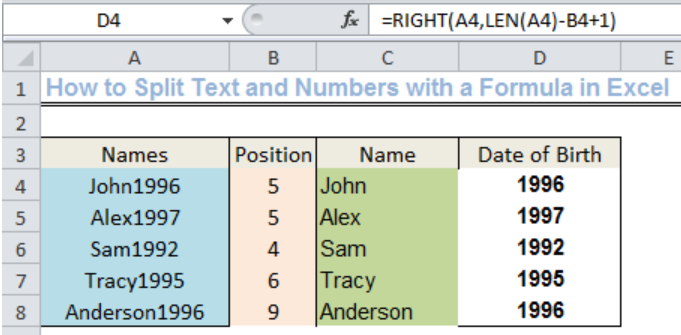Figure 1: Split text and Numbers with a Formula

## Setting up the Data

We will set up the data by inserting the Names of five college students in Column A, including their date of birth without leaving a space between the name and the date of birth.

We will also input Position, Name, and Date of Birth into Cell B3, Cell C3, and Cell D3 respectively.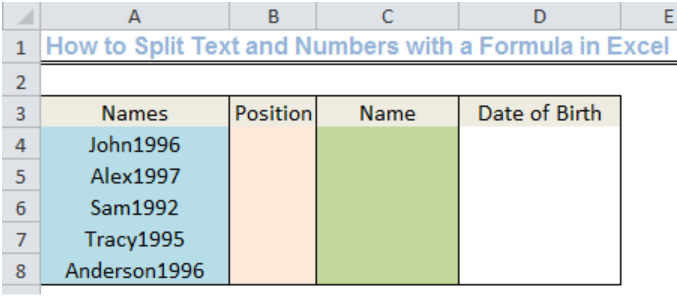Figure 2: Setting up the Data

## The Basic Formula

`=MIN(FIND({0,1,2,3,4,5,6,7,8,9},A1&"0123456789"))`

• We can use the basic formula to split text and numbers from a cell in accordance with the LEFT or RIGHT function as per the requirements.

## Determining the Position of THE FIRST NUMBER in Cell A4 to Cell A8

• We will insert the formula below into Cell B4

`=MIN(FIND({0,1,2,3,4,5,6,7,8,9},A4&"0123456789"))`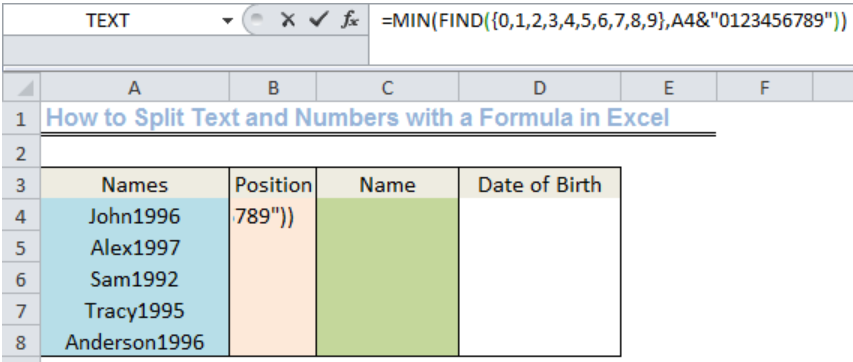Figure 3: Position of the First Number

• Now, we will press enter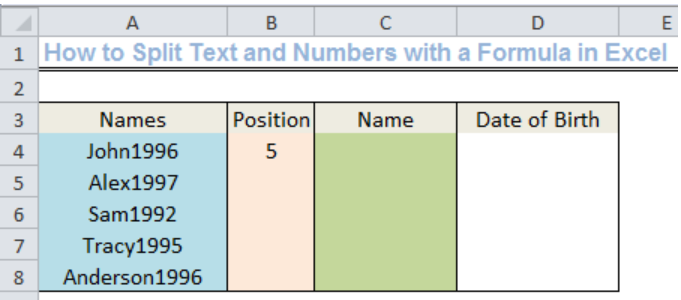Figure 4: Position of the First Number

• As shown in figure 4, this formula returns the position of the first number in Cell A4. JOHN comprises of four letters, the next value is not a text but a number, hence, the position of the first number is 5
• We will use the drop-down feature to get the positions of the first number for the remaining four students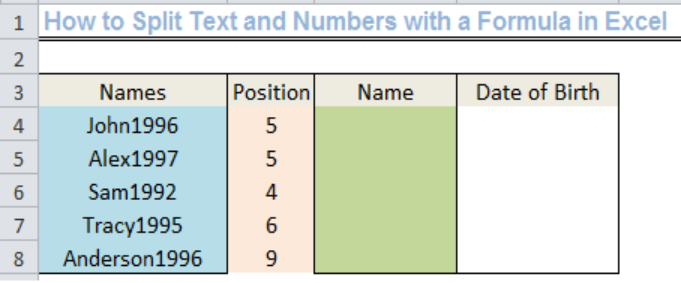Figure 5: Position of the First Number

## Extracting the Text with the LEFT function

• We will use the formula below to extract the text (names) from Column A:

`=LEFT(A4,B4-1)`

• We will insert this formula into Cell C4 and use the drop-down feature to fill the remaining cells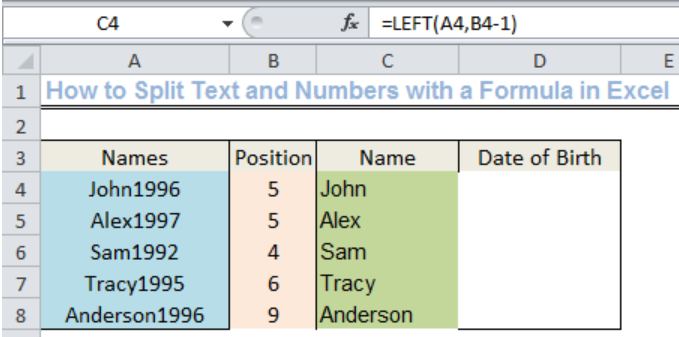Figure 6: Split text and Numbers with the LEFT function

## Extracting the Numbers with the RIGHT function

• We will use the formula below to extract the numbers (date of birth) from Column A:

`=RIGHT(A4,LEN(A4)-B4+1)`

• We will insert this formula into Cell D4 and use the drop-down feature to fill the remaining cells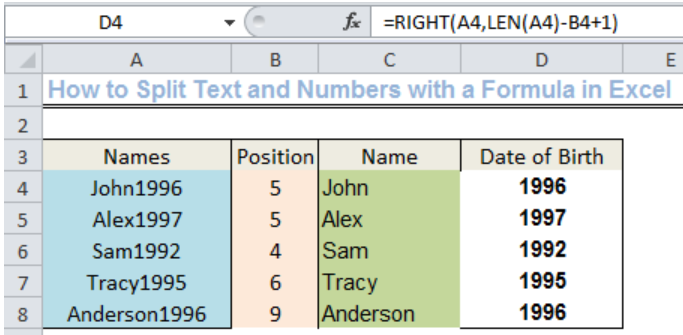Figure 7: Split text and Numbers with the RIGHT function

## Instant Connection to an Expert through our Excelchat Service

Most of the time, the problem you will need to solve will be more complex than a simple application of a formula or function. If you want to save hours of research and frustration, try our live Excelchat service! Our Excel Experts are available 24/7 to answer any Excel question you may have. We guarantee a connection within 30 seconds and a customized solution within 20 minutes.

### Did this post not answer your question? Get a solution from connecting with the expert.Another blog reader asked this question today on Excelchat:
Solution examplesI have text and number in multiple cells (Ex: Cell 1: 4S, Cell 2: 3.5A, Cell 3: 8O) and want to sum the number values only (Ex: 4 + 3.5 + 8). How can I do this?
Solved by O. A. in 60 minsWhat is the formula for splitting up one column text into 2 columns?
Solved by B. H. in 20 minsCan you re edit this formula for me =IFERROR(IF(LEN(MID("RHS",1,FIND("RHS",B4,1)))=1,LEFT(B4,3),MID("RHS",1,FIND("RHS",B4,1))),MID("LHS",1,FIND("LHS",B4,1)))
Solved by S. L. in 40 minsI am having issues creating the one way data table to show the means and standard deviations when you change the proportion of portfolio 1. I have the mean formula and the standard deviation formula at the top and -1 through 1 down the left side. I highlight all of it and create a data table. I reference the "proportion of portfolio 1" cell in the column input box but when the data table comes out it has all the same numbers all they way down the table.
Solved by G. Q. in 49 minsI need to create a formula that extracts the prefix from column A
Solved by I. Q. in 42 mins## Subscribe to Excelchat.coAnother blog reader asked this question today on Excelchat: